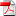# Efficient volatility estimation in a two-factor model - Olivier Féron, Pierre Gruet, and Marc Hoffmann

27
Jun

We statistically analyse a multivariate HJM diffusion model with stochastic volatility. The volatility process of the first factor is left totally unspecified while the volatility of the second factor is the product of an unknown process and an exponential function of time to maturity. This exponential term includes some real parameter measuring the rate of increase of the second factor as time goes to maturity. From historical data, we efficiently estimate the time to maturity parameter in the sense of constructing an estimator that achieves an optimal information bound in a semiparametric setting. We also identify nonparametrically the paths of the volatility processes and achieve minimax bounds. We address the problem of degeneracy that occurs when the dimension of the process is greater than two, and give in particular optimal limit theorems under suitable regularity assumptions on the drift process. We consistently analyse the numerical behaviour of our estimators on simulated and real datasets of prices of forward contracts on electricity markets.

### Download Attachments

•RR-FIME-19-06
File size: 736 KB Downloads: 206